# Using Guess Toggle Levels: An Example

The following is an example of how you could use guess toggle levels to solve a Fathom It! board. The example uses guess toggle levels to keep track of a logical train of thought.

The board position

Our example begins while solving a real-life Fathom It! board. (The board used is Expert Board #3 in the Shareware version, or Expert Board #4 in the registered version.)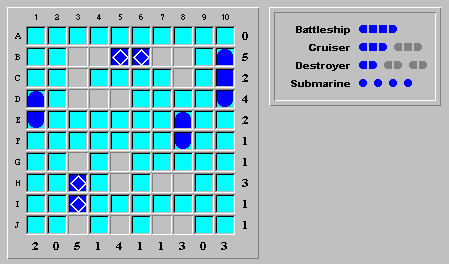A brief examination uncovers the following facts:

· The battleship cannot be placed at (G,3)-(J,3), as this would leave too few empty squares in column 5. Therefore, the battleship belongs somewhere in the sequence (B,3)-(B,8).

· The last remaining cruiser cannot be placed at (B,3)-(D,3), as this would completely close off column 4 with water squares.

· The last remaining cruiser cannot be placed at (D,3)-(D,5). Doing so would force a water square in (C,3), which would force a ship segment in (C,8), which would force a water in (B,7). This would leave nowhere on the board to place the battleship.

The facts above lead us to the conclusion that the remaining cruiser must be placed at either (G,3)-(I,3) or (H,3)-(J,3).

Assume cruiser is at (G,3)-(I,3)

Let's assume the remaining cruiser is positioned at (G,3)-(I,3). Since we are not sure about our assumption, we will toggle the relevant squares, but will not finalize them. Should our assumption prove logically wrong, we'll erase the guesses.

Toggling square (G,3) to be a ship segment infers the following square identities:

1  The rest of row G is water, so (G,5) is water.

2  This means the remaining squares in column 5 are ship segments. Therefore, (D,5) is a ship segment, while (H,5) and (J,5) are submarines.

3  With (J,5) a submarine, the rest of row J is water.

Here's what the board looks like now: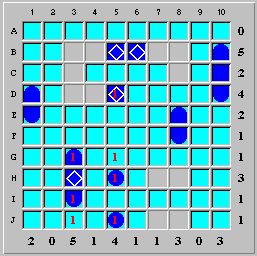You'll notice that the squares displaying our guesses have the numeral "1" (one) inside. These are level one guesses, and are meant to record our initial guesses. They are temporary, are invisible to the automatic solver, and can be erased at any time. It is like writing our guesses in pencil; we may end up erasing the guess if it leads to an inconsistent result.

Let's put it this way: the level one guesses are what the board would look like in answer to the question "what if the remaining cruiser were placed at (G,3)-(I,3)?".

Fork in the logic: up to guess level two!

Now we come to a fork in the road: (D,4) can be either a ship segment or water. Like the cruiser assumption above, we'll toggle (D,4) to a ship segment, toggling other inferred squares. To keep the two assumptions separate, we'll step up to guess toggle level two by pressing CTRL+2. Now, any square toggled will have a numeral "2" inside the square.

Assuming (D,4) is a ship segment infers the following square identities:

1  The remaining squares in row D are water, specifically (D,3).

2  Square (C,3) is water (diagonally adjacent to (D,4)).

Here's what the board looks like, with both level one and level two guesses displayed: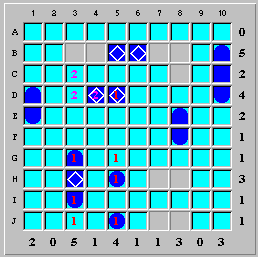Note that column 3 has only one empty square left, but needs two more ship segments. The conclusion is that our last assumption, that square (D,4) is a ship segment, is wrong. Let's erase the guesses in (D,4) and all affected squares by clicking the "Undo Guesses" button on the playing board (or selecting the menu option Guess|Undo Level 2 guesses).

We now see why stepping up to a level two guess is good: it keeps different logical trains of thought separate, enabling you to erase (or backtrack) any one assumption without affecting others.

Continuing the first assumption: (D,4) is water

Let's summarize our logic until now: if the remaining cruiser is placed at (G,3)-(I,3), then (D,4) must be water. Since (D,4)'s being water follows logically from the cruiser placement, we can go back to level one toggling. Press CTRL+1 to reset the guess toggle level to one, and toggle (D,4) to water.

Assuming (D,4) is water infers the following square identities:

1  (B,4) is a ship segment, because column 4 has a tally of one.

2  (C,3) is water, because it is diagonally adjacent to (B,4).

3  (D,3) is a submarine, because row 4 has a tally of four.

Here's what the board looks like now: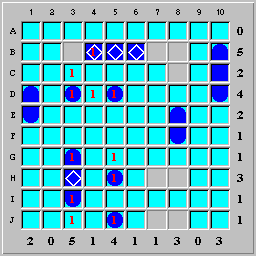Here we hit a contradiction: there are four submarines visible on the board. However, row H must contain a fifth submarine at either (H,7) or (H,8).

Conclusion: cruiser at (H,3)-(J,3)

We've just shown that assuming the cruiser is placed at (G,3)-(I,3) leads to a contradiction: (D,4) can be neither a ship segment nor water. Therefore, our original assumption that the remaining cruiser is placed at (G,3)-(I,3) is wrong: the cruiser is actually positioned at (H,3)-(J,3). Undoing all guesses and finalizing the cruiser at (H,3)-(J,3) leaves us with the following board: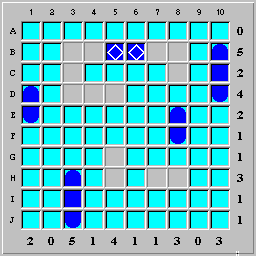Summary: Using guess toggle levels

In the example above, we used guess toggle levels as follows:

1  We attempted to place the remaining cruiser with level one toggles. Inferred squares were also level one toggles.

2  We explored the consequences of (D,4) as a ship segment, using level two toggling. This kept the second assumption separate from the first.

3  When the second assumption proved wrong, we easily removed all level two guesses and returned to level one toggling.

4  When assuming (D,4) to be water also proved wrong, we knew the cruiser had been incorrectly placed.

5  We easily undid all guesses and finalized the cruiser in its correct position.